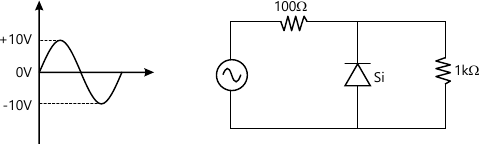MORE IN Basic Electronics Engineering (BXE)
SPPU First Year Engineering (Semester 1)
Basic Electronics Engineering (BXE)
December 2014
Total marks: --
Total time: --
INSTRUCTIONS
(1) Assume appropriate data and state your reasons
(2) Marks are given to the right of every question
(3) Draw neat diagrams wherever necessary

Answer any one question from Q1 and Q2
1 (a) Compare performance of half wave rectifier and full wave rectifier with respect to following parameters:
1) IDC
2) IRMS
3) Rectifier efficiency
4) Ripple factor
5) PIV
6) TUF
6 M
1 (b) Explain how transistor can be used as an amplifier with the help of D.C. load line approach.
6 M

2 (a) Explain the operation of n-channel enhancement type MOSFET with its characteristics.
6 M
2 (b) Determine the O/P waveform for the circuit shown in fig.6 M

Answer any one question from Q3 and Q4
3 (a) Explain the working of inverting summing amplifier with two inputs along with its wave foRMS.
6 M
3 (b) State and prove the de Morgan's theorem. Simplify the following Boolean expression: AB+AB
6 M

4 (a) With the help of block diagram of IC555 explain its operation in Astable mode.
6 M
4 (b) Compare Microprocessor and micro-controller.
6 M

Answer any one question from Q5 and Q6
5 (a) Explain the operation of SCR with the help of V-I characteristics.
7 M
5 (b) Explain the selection criteria of a Transducer.
6 M

6 (a) Define 'Dark current'. Draw and explain the characteristics of photo transistor.
6 M
6 (b) Explain the construction of DIAC and draw its characteristics.
7 M

Answer any one question from Q7 and Q8
7 (a) Draw and explain the electromagnetic or IEEE frequency spectrum.
7 M
7 (b) Compare AM and FM.
6 M

8 (a) Draw and explain the block diagram of GSM.
7 M
8 (b) A carrier of 10 V peak and frequency 100 KHz is amplitude modulated by a sine wave of 4V peak and frequency 1000 Hz. Determine the modulation index for the modulated wave and draw the frequency spectrum for AM wave.
6 M

More question papers from Basic Electronics Engineering (BXE)